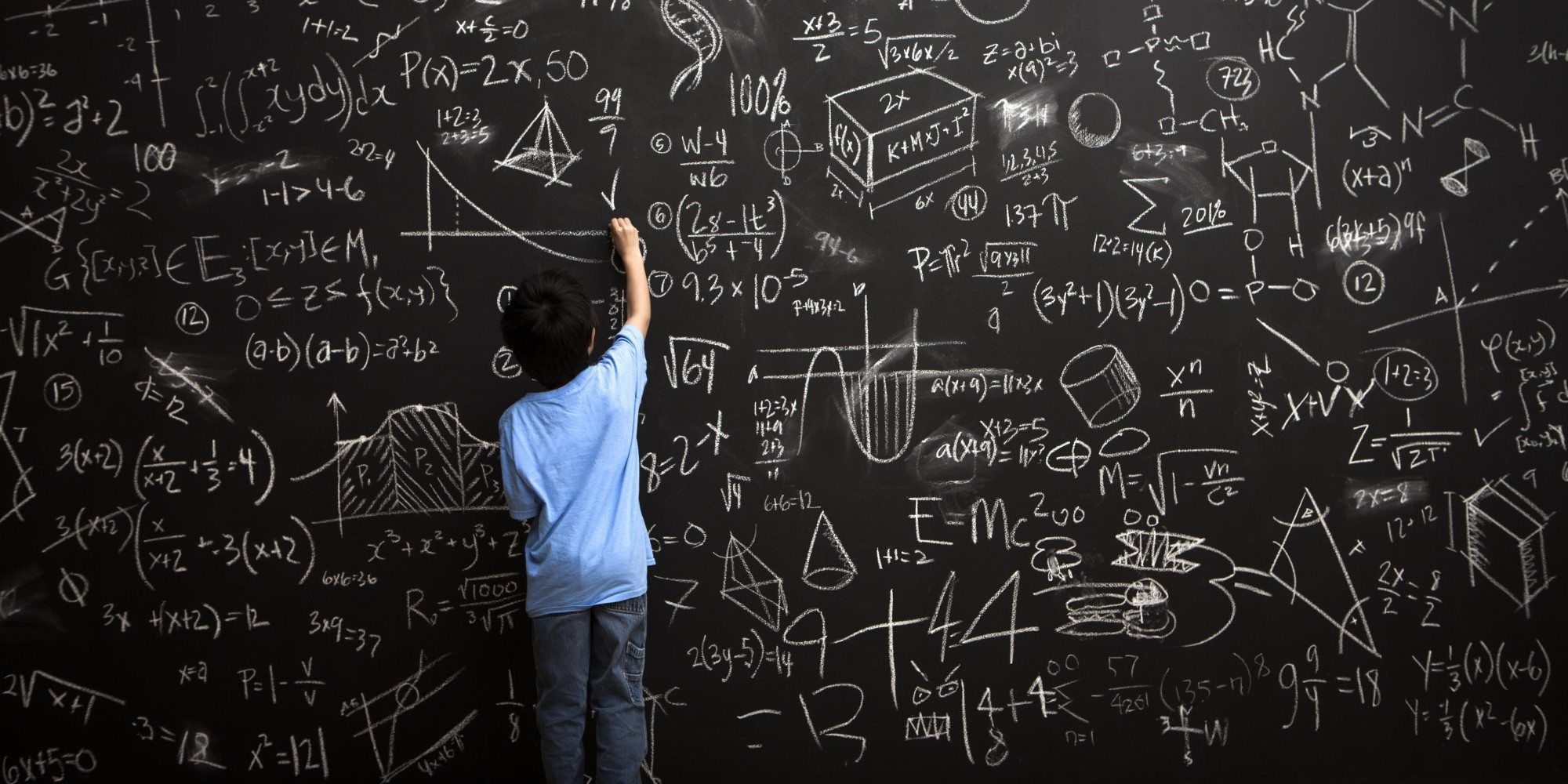## BlogQuantum theory is strange and counterintuitive, but it’s very precise. Lots of analogies and broad concepts are presented in popular science trying to give an accurate description of quantum behavior, but if you really want to understand how quantum theory (or any other theory) works, you need to look at the mathematical details. It’s only the mathematics that shows us what’s truly going on.

Mathematically, a quantum object is described by a function of complex numbers governed by the Schrödinger equation. This function is known as the wavefunction, and it allows you to determine quantum behavior. The wavefunction represents the state of the system, which tells you the probability of various outcomes to a particular experiment (observation). To find the probability, you simply multiply the wavefunction by its complex conjugate. This is how quantum objects can have wavelike properties (the wavefunction) and particle properties (the probable outcome).

No, wait. Actually a quantum object is described by a mathematical quantity known as a matrix. As Werner Heisenberg showed, each type of quantity you could observe (position, momentum, energy) is represented by a matrix as well (known as an operator). By multiplying the operator and the quantum state matrix in a particular way, you get the probability of a particular outcome. The wavelike behavior is a result of the multiple connections between states within the matrix.